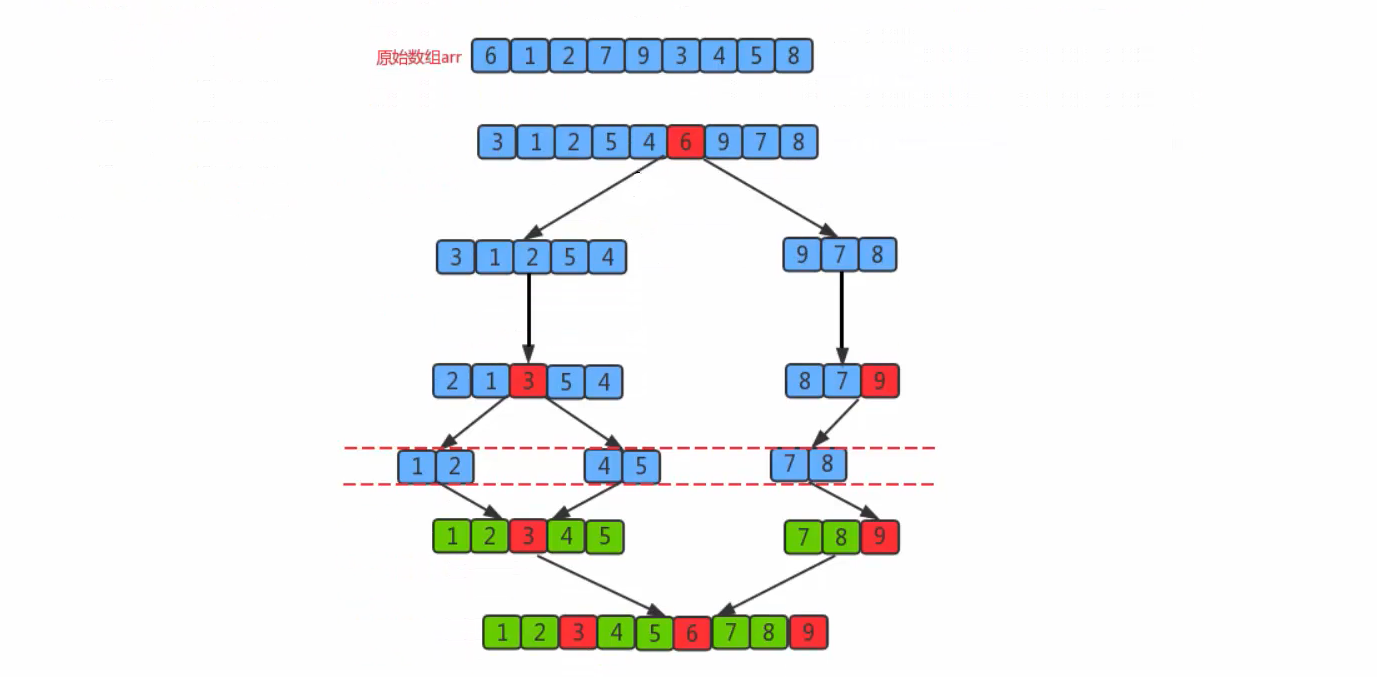### 快速排序(Quick Sort)

xiaohai 2021-06-16 10:26:28 2389人围观 标签： 算法

### 3.6.1、需求

• 排序前：{6, 1, 2, 7, 9, 3, 4, 5, 8}
• 排序后：{1, 2, 3, 4, 5, 6, 7, 8, 9}

### 3.6.2、排序原理

• 首先确定一个分界值，通过该分界值将数组分成左右两个部分
• 将大于或等于分界值的数据放在右边，小于分界值的放在左边，此时左边部分中各个部分都小于或等于分界值，而右边部分中的各个元素都大于或等于分界值
• 然后左边和右边的数据可以独立排序，按照上一步的操作继续这样分组，这样就可以对数据进行排序### 3.6.3、API设计

private static void sort(Comparable[] a,int lo,int hi)：对数组a中从索引lo到索引hi之间的元素进行排序
private static void partition(Compareble[] a,int lo,int hi)：对数组中a，从索引lo到hi之间的元素进行分组，并返回分组界限值对应的索引
private static boolean grater(Comparable v,Comparable w)：判断v是否大于w
private static void exch(Comparable[] a,int i,int j)：交换a数组中，索引i和索引j处的值

### 3.6.4、代码实现

``````package cn.test.algorithm.sort;

public class Quick {
/**
* 对数组元素进行排序
*
* @param a
*/
public static void sort(Comparable[] a) {
int lo = 0;
int hi = a.length - 1;

sort(a, lo, hi);
}

//对数组中lo到hi索引处的元素进行排序
private static void sort(Comparable[] a, int lo, int hi) {
//安全校验
if (hi <= lo) {
return;
}

//需要对数组中lo索引到hi索引的元素进行分组
int partition = partition(a, lo, hi);
//让左子组有序
sort(a, lo, partition - 1);
//让右子组有序
sort(a, partition + 1, hi);
}

//对数组a中，从索引lo到hi之间的元素进行分组，并返回分组界限对应的索引
public static int partition(Comparable[] a, int lo, int hi) {
//定义分界值，选择数组的第一个元素，就是lo这个
Comparable value = a[lo];

//定义两个指针，左边指向数组的最左边，右边指向数组的最后边+1
int left = lo;
int right = hi + 1;

//进行遍历
while (true) {
//首先从最右边开始搜索，找到比分界值小的元素
while (greater(a[--right], value)) {
if (right == lo) {//如果一直遍历都没有找到这个数，那么就需要跳出循环
break;
}
}

//再次从最左边开始搜索，找到比分界值大的元素
while (greater(value, a[++left])) {
if (left == hi) {//如果一直遍历都没有找到这个数，也需要跳出循环
break;
}
}

//停止搜索，left和right相等的时候
if (left >= right) {
exch(a, lo, right);//最终还需要交换一次
break;
} else {
exch(a, left, right);//交换数据
}
}

return right;
}

/**
* 比较v元素是否大于w元素
*
* @return
*/
private static boolean greater(Comparable v, Comparable w) {
return v.compareTo(w) > 0;
}

/**
* 交换i和j处的元素值
*
* @param a
* @param i
* @param j
*/
private static void exch(Comparable[] a, int i, int j) {
Comparable temp;
temp = a[i];
a[i] = a[j];
a[j] = temp;
}
}
``````

``````package cn.test.algorithm.test;

import cn.test.algorithm.sort.Quick;

import java.util.Arrays;

public class TestQuick {
public static void main(String[] args) {
Integer[] arr = {6, 1, 2, 7, 9, 3, 4, 5, 8};
Quick.sort(arr);
System.out.println(Arrays.toString(arr));
}
}
``````

``````[1, 2, 3, 4, 5, 6, 7, 8, 9]
``````

### 3.6.6、时间复杂度分析

• 最优情况：每次切分选择的基准数字刚好将当前序列等分
• 如果我们把数组的切分看做是一个树，那么需要切分log(n)次，所以最优情况下快速排序的时间复杂度为O(nlog(n))
• 最坏情况：每一次切分选择的基准数字是当前序列中的最大值或最小值，这使得每一次切分都会有一个子组，那么共需要切分n次，所以最坏的情况时间复杂度为O(n^2)
• 平均情况：每一次切分的基准数字不是最大值和最小值，也不是中值，这种情况可以使用数学归纳法证明，快速排序的时间复杂度为O(nlog(n))。